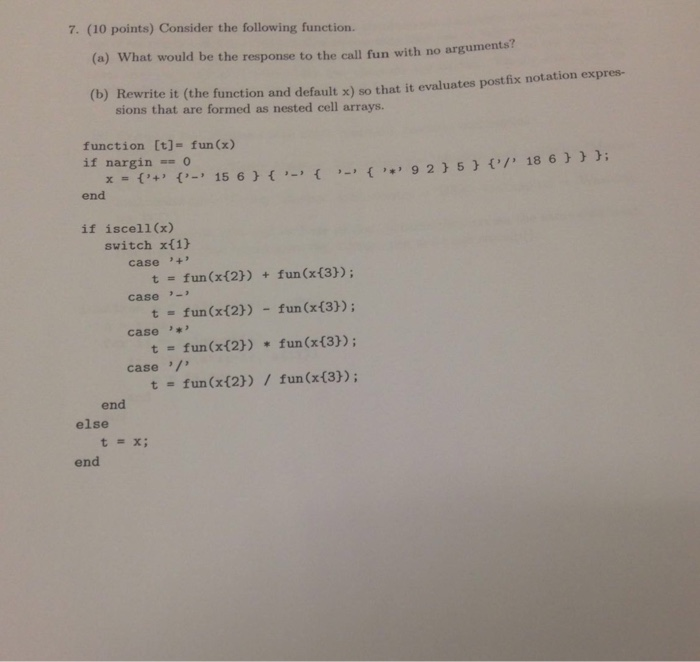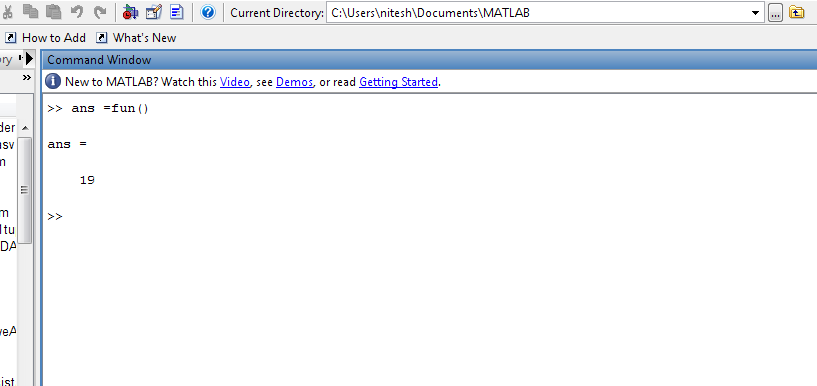# Question & Answer: Consider the following function. (a) What would be the response to the call fun with no arguments? (b) Rewrite it (the function an…..Consider the following function. (a) What would be the response to the call fun with no arguments? (b) Rewrite it (the function and default x) so that it evaluates post fix notation expressions that are formed as nested cell arrays. function [t] = fun(x) if nargin == 0 x = { ‘+’ { ‘-‘ { ‘*’ 9 2 } 5} { ‘/’ 18 6 } } }: end if iscell(x) switch x(1) case ‘+’ t = fun (x{2}) + fun (x{3}): case ‘-‘ t = fun (x{2}) – fun (x{3}): case ‘*’ t = fun(x{2}) * fun (x{3}): case ‘/’ t = fun (x{2})/fun (x{3}): end else t = x: end

[a]

Don't use plagiarized sources. Get Your Custom Essay on
Question & Answer: Consider the following function. (a) What would be the response to the call fun with no arguments? (b) Rewrite it (the function an…..
GET AN ESSAY WRITTEN FOR YOU FROM AS LOW AS \$13/PAGE

Code :

function [t] = fun(x)

if nargin ==0

x = {‘+’ {‘-‘ 15 6} {‘-‘ {‘-‘ {‘*’ 9 2} 5 } {‘/’ 18 6}}};

end

if iscell(x)

switch x{1}

case ‘+’

t= fun(x{2}) + fun(x{3});

case ‘-‘

t = fun(x{2}) – fun(x{3});

case ‘*’

t = fun(x{2}) * fun(x{3});

case ‘/’

t = fun(x{2}) / fun(x{3});

end

else

t =x;

end

Result :